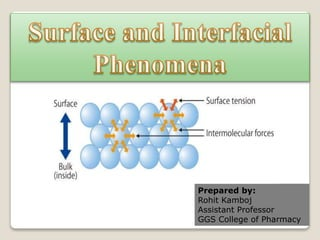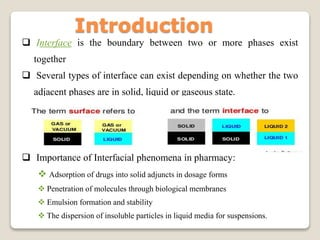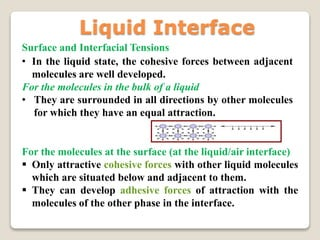Ce diaporama a bien été signalé.
Le téléchargement de votre SlideShare est en cours. ×

# surfaceandinterfacialphenomena-200417163249.pdf

Publicité
Publicité
Publicité
Publicité
Publicité
Publicité
Publicité
Publicité
Publicité
Publicité
PublicitéChargement dans…3
×

## Consultez-les par la suite

1 sur 31 Publicité

# surfaceandinterfacialphenomena-200417163249.pdf

It covers all the topics of surface tension and interfacial tension from physical Pharmaceutics-I 3rd Semester.

It covers all the topics of surface tension and interfacial tension from physical Pharmaceutics-I 3rd Semester.

Publicité
Publicité

### surfaceandinterfacialphenomena-200417163249.pdf

1. 1. Prepared by: Rohit Kamboj Assistant Professor GGS College of Pharmacy
2. 2. Introduction  Interface is the boundary between two or more phases exist together  Several types of interface can exist depending on whether the two adjacent phases are in solid, liquid or gaseous state.  Importance of Interfacial phenomena in pharmacy:  Adsorption of drugs into solid adjuncts in dosage forms  Penetration of molecules through biological membranes  Emulsion formation and stability  The dispersion of insoluble particles in liquid media for suspensions.
3. 3. Surface and Interfacial Tensions • In the liquid state, the cohesive forces between adjacent molecules are well developed. For the molecules in the bulk of a liquid • They are surrounded in all directions by other molecules for which they have an equal attraction. For the molecules at the surface (at the liquid/air interface)  Only attractive cohesive forces with other liquid molecules which are situated below and adjacent to them.  They can develop adhesive forces of attraction with the molecules of the other phase in the interface. Liquid Interface
4. 4. Surface Tension  Thus SURFACE TENSION [γ ] is the force per unit length that must be applied parallel to the surface so as to counterbalance the net inward pull and has the units of dyne/cm.  INTERFACIAL TENSION is the force per unit length existing at the interface between two immiscible liquid phases and has the units of dyne/cm.  If two liquids are completely miscible, no interfacial tension exists between them.
5. 5. • Internal Factors : The surface tension is observed in liquids due to Intermolecular attractive forces. • External Factors : When substances are added to liquids, these alter the surface and interface tension. Example are Surface active agents decreases Surface tension • Effect of Temperature: As temp increases the Surface tension of liquids decreases due to enhance kinetic energy that weakens cohesive force. At critical temp Surface tension is 0. Factors influencing Surface and Interfacial tension
6. 6.  Methods for measuring surface and interfacial tension 1- Capillary rise method 2- Ring (Du Nouy) tensiometer 3- Drop weight method (Stalagmometer) 4- Drop Count Method  The choice of the method for measuring surface and interfacial tension depend on:  Whether surface or interfacial tension is to be determined.  The accuracy desired  The size of sample.
7. 7. 7 Capillary Rise Method When a capillary tube is placed in a liquid, it rises up the tube a certain distance. By measuring this rise, it is possible to determine the surface tension of the liquid. It is not possible, to obtain interfacial tensions using the capillary rise method.  Cohesive force is the force existing between like mole- cules in the surface of a liquid Adhesive force is the force existing between unlike molecules, such as that between a liquid and the wall of a glass capillary tube  When the force of Adhesion is greater than the cohesion, the liquid is said to wet the capillary wall, spreading over it, and rising in the tube. The Principle
8. 8. 8 The upward component of the force resulting from the surface tension of the liquid at any point on the circumference is given by: Thus the total upward force around the inside circumference of the tube is Where Ө = the contact angle between the surface of the liquid and the capillary wall 2 π r = the inside circumference of the capillary. For water the angle Ө is insignificant, i.e. the liquid wets the capillary wall so that cos Ө = unity Cont. angle water and glass a = γ cos Ө a = 2 π r γ cos Ө
9. 9. 9 The downward force of gravity Downward component b = (mass x acceleration) b = (mass x acceleration + w) = Volume x density x acceleration = cross-sectional area x height x density x acceleration At Maximum height, the opposing forces are in equilibrium i.e. a=b Where: h = the height of the liquid column to the lowest point of the meniscus p = density of the liquid g = the acceleration of gravity w = the weight of the upper part of the meniscus. 2 π r γ = π r 2 h p g γ = 1/2 r h p g π r 2 x h x p x g
10. 10. Ring (Du Nouy) Tensiometer  the principle of the instrument depends on the fact that: the force necessary to detach a platinum-iridium ring immersed at the surface or interface is proportional to the surface or interfacial tension.  The force of detachment is recorded in dynes on a calibrated dial  The surface tension is given by: Where: F = the detachment force R1 and R 2= the inner and outer radii of the ring. γ = F / 2 π (R1 + R2)  For measuring surface and interfacial tensions. The principle
11. 11. 11 If the volume or weight of a drop as it is detached from a tip of known radius is determined, the surface and interfacial tension can be calculated from Where m = the mass of the drop V = the volume of the drop p = the density of the liquid r = the radius of the tip g = the acceleration due to gravity  The tip must be wetted by the liquid so as the drop doesn’t climb the outside of the tube. γ1 = vρ1g 2 π rn¹ Drop Weight and Drop volume method γ2 = vρ2g 2 π rn2 Drop Count γ1/γ2 = ρ1n2/ρ2n1 Drop Weight γ1/γ2 = W1/W2
12. 12. 12 When a liquid such as oleic acid is placed on the surface of other liquid like water, it will spread as a film if the adhesion force is greater than the cohesive forces. The term film applies to a duplex film as opposed to monomolecular film. Duplex films are sufficiently thick so that surface and interface are independent of one another. Spreading coefficient γ L γ S γ Ls
13. 13. 13 As surface or interfacial work is equal to surface tension multiplied by the area increment. The work of cohesion, which is the energy required to separate the molecules of the spreading liquid so as it can flow over the sub-layer= Where 2 surfaces each with a surface tension = γ L The work of adhesion, which is the energy required to break the attraction between the unlike molecules = Where: γ L =the surface tension of the spreading liquid γ S =the surface tension of the sublayer liquid γ LS =the interfacial tension between the two liquids. Spreading occurs if the work of adhesion is greater than the work of cohesion, i.e. Wa > Wc or Wa - Wc > 0 Wc = 2 γ L Wa = γ L + γ S - γ LS
14. 14. Wc = 2 γ L Wc = γLΔA + γLΔA S= WA- Wc Wc = 2 γLΔA ΔA=1cm Work of Cohesion Wc = 2γL-----1 γ L γ L Wa = γ L ΔA + γ S ΔA - γ LSΔA Work of Adhesion Wa = γ L + γ S - γ LS ------2 γ L γ S
15. 15. 15 Spreading Coefficient is The difference between the work of adhesion and the work of cohesion S = Wa - Wc = (γ L + γ S - γ LS ) - 2 γ L S = γ S - γ L - γ LS S = γ S – (γ L + γ LS ) Spreading occurs (S is positive) when the surface tension of the sub-layer liquid is greater than the sum of the surface tension of the spreading liquid and the interfacial tension between the sub-layer and the spreading liquid. If (γ L + γ LS ) is larger than YS , (S is negative) the substance forms globules or a floating lens and fails to spread over the surface.
16. 16. 16 Application of Spreading coefficient in pharmacy  The requirement of film coats to be spreaded over the tablet surfaces.  The requirement of lotions with mineral oils to spread on the skin by the addition of surfactants.
17. 17. 17
18. 18. ADSORPTION:  It is surface effect.  E.g. concentration of alkaloid molecule on the surface of clay. ABSORPTION:  Gas or liq penetrates in to the capillary spaces of absorbing medium.  The taking up of water by a sponge is absorption. 18
19. 19.  Positive adsorption: The concentration of dissolved material on the interface is higher than that of solution internal.  Negative adsorption: The concentration of dissolved material on the interface is less than that of solution internal. 19
20. 20. 20  Molecules and ions that are adsorbed at interfaces are termed surface active agents, surfactants or amphiphile  The molecule or ion has a certain affinity for both polar and nonpolar solvents.  Depending on the number and nature of the polar and nonpolar groups present, the amphiphile may be hydrophilic, lipophilic or be reasonably well-balanced between these two extremes.  It is the amphiphilic nature of surface active agents which causes them to be adsorbed at interfaces, whether these be liquid/gas or liquid/liquid.
21. 21. Surface active agent
22. 22. 22 Functional Classification According to their pharmaceutical use, surfactants can be divided into the following groups:  Wetting agents  Solubilizing agents  Emulsifying agents  Dispersing, Suspending and Defloculating agents  Foaming and antifoaming agents  Detergents
23. 23. 23 A scale showing classification of surfactant function on the basis of HLB values of surfactants. The higher the HLB of a surfactant the more hydrophilic it is. Example: Spans with low HLB are lipophilic. Tweens with high HLB are hydrophilic.
24. 24. Adsorption at Solid interface  A gas or liquid is adsorbed on solid surface.  The material used to adsorb gas or liquid (solid) is known as adsorbent.  The substance that is attached to the surface of the solid is called adsorbate.  The degree of adsorption of gas by a solid depends on 1. Nature of adsorbent and its surface area. 2. Nature of adsorbate and the partial pressure of gas. 3. Temp. 24
25. 25. Type of adsorption. Physical adsorption Chemical adsorption Reversible Irreversible Weak van der Waals forces Strong chemical bond Non-specific More specific Common at low temp Occurs at high temp Heat of adsorption is low (20 to 40 kj/mole) Heat of adsorption is high (40 to 400 kj/mole) e.g. adsorption of gases on charcoal e.g. adsorption of oxygen on silver or gold. 25 Combination of both type of adsorption is known as sorption. Desorption : adsorbed molecules or ions are removed from the solid surface.
26. 26. Adsorption at solid/gas interface  Adsorption of gas is important in following area 1. Removal of objectionable odors from the room. 2. Prevention of obnoxious gases entering in to the body (gas masks) 3. Estimation of surface area and particle size of powders.  The amount of gas adsorbed per unit area or unit mass of solid is measured at diff pressure of the gas at constant temp.  The graph of amt of gas ad./unit A or m of solid Vs pressure is known as adsorption isotherm. 26
27. 27. Freundlich isotherm  The relationship between pressure of the gas and amt adsorbed at constant temp has been expressed by freundlich isotherm eqn:  Where x = wt of gas adsorbed per unit wt of adsorbent,m  P= equilibrium pressure, k and n = constant.  This eqn gives curvilinear graph when (x/m) is plotted against pressure p.  The constant k and n are evaluated from the exp and they depends on temp and nature of the adsorbent and adsorbate. 27 n kP m x y / 1  
28. 28. Freundlich isotherm 28 x/m p Log (x/m) Log p Slope = 1/n Intercept = k
29. 29. Langmuir Adsorption  The following assumptions are made for this: 1. The surface of solid posses fixed number of active sites for the adsorption of gases. 2. At max adsorption, the gas layer that is found around the solid is of only one molecule thick. 3. The rate of adsorption (condensation) is proportional to number of sites unoccupied. 4. The rate of evaporation (desorption) is proportional to the number of occupied sites. 29
30. 30. Langmuir Adsorption  The adsorption of gas on the solid surface depends on the pressure of the gas in the experimental conditions.  At a particular pressure, p,  Fraction of site occupied : Ө  Fraction of sites unoccupied: (1-Ө)  Rate of adsorption: r1 = k1(1-Ө)P,  Rate of desorption: r2 = k2Ө,  Where k1 and k2 are proportionality constants for the process of adsorption and desorption. 30
31. 31.  At equilibrium : r1 = r2  If we consider,  y= mass of gas adsorbed/g of adsorbrnt  ym= mass of gas that 1 g of adsorbent can take up when a monolayer is complete. 31 ) 1 2 ( 1 1 ) 1 2 ( 2 1 1 2 ) 1 ( 1 P k k P k P k P k k k P k P k k P k                 